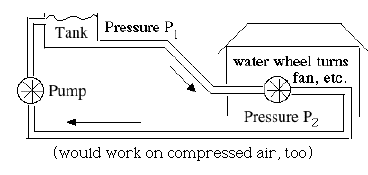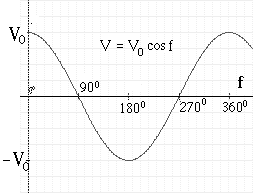(E10)   Alternating Current (AC)

 Note:   This section and others concerned with alternating current require an understanding of sine and cosine mathematical functions, resembling the outlines of waves. They are discussed in the sections on trigonometry, starting with "(M-7) Trigonometry---What is it good for?," though the continuous sine wave is only introduced in (M-10).

In a flashlight the electric current always flows in the same direction. By an old convention dating back to Ben Franklin the flow is viewed as going from the positive (+) end of the battery, through the electrical load (e.g. lightbulb) whose resistance limits the intensity of the current, to the negative (–) end, closed by a return current inside the battery, which also generates the voltage by a chemical process. We call such a single-direction flow direct current or DC in short.

(The side we call "positive" differs from the "negative" one by the chemistry inside the battery. If we used plates of zinc and copper dipping in acid, as early batteries did, the zinc side would be called negative. The labeling of (+) and (–) follows a guess by Ben Franklin, who only knew static electricity: today we know that the electrons which carry the current have negative charge and flow from the (–) to the (+), but it is much too late to change notations and convention.)

The current flowing from outlets in the home is of a completely different kind. In the US it reverses direction 120 times each second, or 60 back-and-forth cycles each second (50 in Europe). That sort is known as alternating current or AC. It is not any harder for power stations to produce AC (indeed, it may be easier) and as will be explained, it is much more economical to send AC to greater distances than to send DC. We will try to describe AC here using the water flow analogy. The analogy may be somewhat strained (skip this part if you wish) but then again, it is just a help to understanding.

The flow of DC in a wire resembles a one-directional flow of water in a pipe. To simulate AC, imagine that the pump wheel which drives the flow, instead of rotating in a constant direction, swirls back and forth like the agitator in a top-load washing machine (fluid and pump are both just an analogy; the sloshing of the electric fluid can be very fast, even at 60 cycles a second, without encountering inertia). The fluid will accordingly slosh back and forth at the same frequency (drawing).That is definitely NOT a good way to deliver water to users, but with electricity what is being delivered is not the fluid but its energy. If, in the location where we want to use that energy, we place a wheel driven back and forth by the flow, then a ratchet wheel can extract constant rotation from the back-and-forth motion--a wheel with sloping gears which only turns in one direction, being stopped by a lever ("pawl") from turning in the opposite direction.

AC flow is somewhat like this. If you are comfortable with trigonometric functions, you might note that the voltage V and the current I both vary in wave-like fashion, like

I = I0 sin f
V = V0 sin f
where the angle f ("phase") varies with time t in seconds like

f = 60 t × 360°

The above relation between f and t shows that in each 1/60 second, f goes through a complete cycle of 360°, and I and V both complete a full wave cycle, like this:The value of I in any direction varies between a maximum I0 (the "amplitude" of the AC current) and –I0, a maximum current in the opposite direction. At the same time the voltage V varies between V0 (the "amplitude" of the AC voltage) and –V0,

Ohm's law still holds, at least if the circuit only includes resistances (when capacitors and magnetic coils are also included, or machinery which uses them, the calculation gets more complex). At any instant
I = V / R

with R the same value as the one you would obtain with DC. However, because both I and V fluctuate rapidly, so does the power delivered

P = I V

What is more significant (and is measured by the wattmeters which determine your electric bill) is not the instantaneous power, which can be anywhere between zero and I0V0, but the average power delivered, which turns out to be in this case 0.5 I0V0.

The peak of the voltage V obtained from wall outlets is not 110 volts, but is larger by a factor equal the square root of 2, about 1.4142...., which makes it about

110 ×1.4142 = 155.6 volts

Similarly, if we connect to the outlet a resistance R, the peak current I0 is V0/R = 155.6/R. The average power however is

P = 0.5 I0V0 = 0.5 V022 / R

because the factor (1.4142..) 2 = 2 cancels exactly the factor 0.5 . In technical terms, if 155.6v is the peak voltage, then 110v is the r.m.s. ("root mean square") voltage, the DC voltage which gives the same amount of power.

To remember:
 The electric current drawn from a battery is direct current (DC), analogous to the steady flow of water in a pipe, in a single direction.     The electric current used in homes and in most commerce is alternating current (AC), analogous to a flow of water in a pipe which rapidly sloshes back and forth. With our water supply at home, we want to actually remove some water and use it; but with electricity, all we usually need is extract some energy, and AC can do this--it can for instance rotate a ratchet wheel.     The current I and voltage V in a simple AC circuit rise and fall in wavelike fashion: I = I0 sin f         V = V0 sin f where "sin f" is the wave-like trigonometric "sine" function. It goes through a complete up-and-down cycle each time the "phase" f goes from 0 to 360° (or more generally, increases by 360°) which in AC current in the USA happens 60 times each second (50 times in the power grids of other countries).     If the circuit only includes resistances, Ohm's law I = V/R is still satisfied, both instantaneously and on the average. The electric power at the peak of the cycle is also still W = I0 V0 but the average electric power is only 0.5 I0 V0 . We say the voltage in the home is 110 volts, but that is actually an average value, the voltage of a DC circuit which (given some resistance R) delivers the same power. The peak voltage V0 is actually 155.6 volts, or 1.4142 times (square root of 2) larger.

 Next Stop: E11.   The Reason for using AC Home Page "All Things Electric and Magnetic"             "From Stargazers to Starships" Timeline of "All Things Electric and Magnetic" Timeline (from "Stargazers") Master index file Author and Curator:   Dr. David P. Stern      Mail to Dr.Stern:   stargaze("at" symbol)phy6.org . Last updated: 13 March 2010